# Marginal distribution

The distribution of a random variable, or set of random variables, obtained by considering a component, or subset of components, of a larger random vector (see Multi-dimensional distribution) with a given distribution. Thus the marginal distribution is the projection of the distribution of the random vector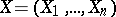onto an axisor subspace defined by variables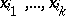, and is completely determined by the distribution of the original vector. For example, if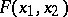is the distribution function ofin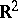, then the distribution function ofis equal to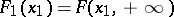; if the two-dimensional distribution is absolutely continuous and if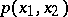is its density, then the density of the marginal distribution of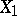is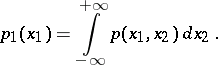The marginal distribution is calculated similarly for any component or set of components of the vectorfor any. If the distribution ofis normal, then all marginal distributions are also normal. Whenare mutually independent, then the distribution ofis uniquely determined by the marginal distributions of the componentsof: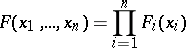and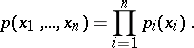The marginal distribution with respect to a probability distribution given on a product of spaces more general than real lines is defined similarly.